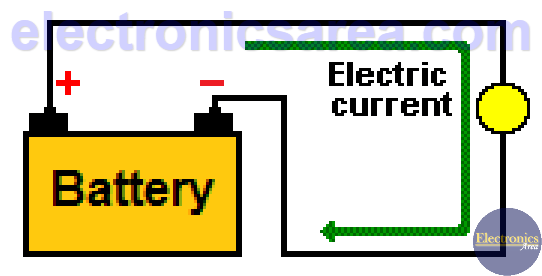# What is a Resistor?

resistor is an electrical element that causes opposition to the flow of the electric current.  Using the Ohm’s Law V = IxR we can find the voltage on the resistors terminals. The diagram below shows an incandescent light bulb, located on the path of an electric current.This current leaves the positive terminal of the battery and returns to the negative terminal, passing through the light bulb. This light bulb behaves like a resistor. Resistors are represented with the capital letter “R” and its values (resistance) are measured in Ohms (Ω).

Resistors are manufactured in a wide variety of values. There are resistors with values of KiloOhms (KΩ), MegaOhms (MΩ). Larger resistors (external dimensions) are those that can dissipate more heat. The larger the resistor, the more heat it can dissipate. Kilo Ohms (KΩ) and MegOhms (MΩ) are used to represent very big resistances. On the following chart we see the equivalences among them.• 1 MicroOhm = 1 x 10-6 Ohms (Ω).
• 1 KiloOhm (KΩ) = 1 x 103 Ohms.
• 1 MegaOhm (MΩ) = 1 x 106 Ohms.
• 1 MegaOhm (MΩ) = 1 x 103 KiloOhms (KΩ).

In order to know the value of a resistor without measuring it, there is a resistor color code that helps us to easily obtain its resistance. To obtain the resistance of any element of a specific material, it is necessary to know some details of the material such as: its length, cross area, specific resistance or resistivity of the material that is made.

## Resistance and Conductance

The conductance measures the ability of a material to allow electrical current to pass and it is measured in Siemens. The reciprocal or inverse of the resistance is the conductance, and it is generally represented by the capital letter “G”. A circuit with high conductance has low resistance, and vice versa. G = 1/R, R = 1/G.

• A resistance of 1 Ohm (Ω) has a conductance of 1 siemens (S).
• A resistance of 1000 Ohms have a conductance of 0.001 siemens.
• A kilosiemens (1 kS) is equal to one thousand (103) siemens.
• A megasiemens (1 MS) is equal to one million (106) siemens.
• A millisiemens (1 mS) is equal to one-thousandth (10-3) of a siemens.
• A microsiemens (1 uS) is equal to one-millionth (10-6) of a siemens.

Note: 1 siemens = 1 mho (“ohm” spelled backwards)

• 2
•
•
•
•
•
•
•
•
2
Shares
•
2
Shares
• 2
•
•
•
•
•
•
•